## Main

Lattice field theories (LFTs) have been instrumental in uncovering a wide range of fundamental physical phenomena, from quark confinement in atomic nuclei20 and neutron stars21 to topologically protected states of matter22 and transport in novel magnetic23 and electronic24,25 materials. LFTs can be constructed either by discretizing the spacetime continuum underlying classical and quantum field theories20, or by approximating discrete physical quantities, such as the electron spins in a crystal lattice, through continuous variables. In equilibrium thermodynamics, LFT approaches have proved invaluable both computationally and analytically, for a single LFT often represents a broad class of microscopically distinct physical systems that exhibit the same universal scaling behaviours in the vicinity of a phase transition4,26. However, until now there has been little evidence as to whether the emergence of order in living matter can be understood within this universality framework. Our combined experimental and theoretical analysis reveals a number of striking analogies between the collective cell dynamics in bacterial fluids and known phases of condensed-matter systems, thereby implying that universality concepts may be more broadly applicable than previously thought.

To realize a microbial non-equilibrium LFT, we injected dense suspensions of the rod-like swimming bacterium Bacillus subtilis into shallow polydimethyl siloxane (PDMS) chambers in which identical circular cavities are connected to form one- and two-dimensional (2D) lattice networks (Fig. 1, Supplementary Fig. 6 and Methods). Each cavity is 50 μm in diameter and 18 μm deep, a geometry known to induce a stably circulating vortex when a dense bacterial suspension is confined within an isolated flattened droplet15. For each cavity i, we define the continuous vortex spin variable Vi(t) at time t as the total angular momentum of the local bacterial flow within this cavity, determined by particle imaging velocimetry (PIV) analysis (Fig. 1b, f; Supplementary Movies 1 and 2 and Methods). To account for the effect of oxygenation variability on suspension motility9, flow velocities are normalized by the overall root-mean-square (r.m.s.) speed measured in the corresponding experiment. Bacterial vortices in neighbouring cavities interact through a gap of predetermined width w (Fig. 1f). To explore different interaction strengths, we performed experiments over a range of gap parameters w (Methods). For square lattices, we varied w from 4 to 25 μm and found that for all but the largest gaps, ww ≈ 20 μm, the suspensions generally self-organize into coherent vortex lattices, exhibiting domains of correlated spins whose characteristics depend on coupling strength (Fig. 1a, e). If the gap size exceeds w, bacteria can move freely between cavities and individual vortices cease to exist. Here, we focus exclusively on the vortex regime w < w and quantify preferred magnetic order through the normalized mean spin–spin correlation χ = 〈ΣijVi(t)Vj(t)/Σij|Vi(t)Vj(t)|〉, where Σij denotes a sum over pairs {i, j} of adjacent cavities and 〈〉 denotes time average.Figure 1: Edge currents determine antiferromagnetic and ferromagnetic order in a square lattice of bacterial vortices.

Square lattices reveal two distinct states of preferred magnetic order (Fig. 1a, e, i), one with χ < 0 and the other with χ > 0, transitioning between them at a critical gap width wcrit ≈ 8 μm (Fig. 1j). For subcritical values w < wcrit, we observe an antiferromagnetic phase with anti-correlated (χ < 0) spin orientations between neighbouring chambers on average (Fig. 1a and Supplementary Movie 1). By contrast, for w > wcrit, spins are positively correlated (χ > 0) in a ferromagnetic phase (Fig. 1e and Supplementary Movie 2). Noting that the r.m.s. spin 〈Vi(t)21/2 decays only slowly with increasing gap width ww (Fig. 1k), and that the chambers do not impose any preferred handedness on the vortex spins (Supplementary Fig. 1 and Supplementary Section 1), we conclude that the observed phase behaviour is caused by spin–spin interactions. However, although both phases possess a well-defined average vortex–vortex correlation, the individual spins fluctuate randomly over time as ordered domains split, merge and flip (Fig. 1i and Supplementary Figs 1 and 3) while the system explores configuration space inside a statistical steady state (Supplementary Sections 1 and 3). Thus, although the bacterial vortex spins {Vi(t)} define a real-valued lattice field, the phenomenology of these continuous bacterial spin lattices is qualitatively similar to that of the classical 2D Ising model4 with discrete binary spin variables si {±1}, whose configurational probability at finite temperature T = (kBβ)−1 is described by a thermal Boltzmann distribution exp(− βJΣijsisj), where J > 0 corresponds to ferromagnetic and J < 0 to antiferromagnetic order. The detailed theoretical analysis below shows that the observed phases in the bacterial spin system can be understood quantitatively in terms of a generic quartic LFT comprising two dual interacting lattices. The introduction of a double lattice is necessitated by the microscopic structure of the underlying bacterial flows. By analogy with a lattice of interlocking cogs, one might have intuitively expected that the antiferromagnetic phase would generally be favoured, because only in this configuration does the bacterial flow along the cavity boundaries conform across the inter-cavity gap, avoiding the potentially destabilizing head-to-head collisions that would occur with opposing flows (Fig. 1b, c). However, the extent of the observed ferromagnetic phase highlights a competing biofluid-mechanical effect.

Just as the quantum Hall effect17 and the transport properties of graphene18,19 arise from electric edge currents, the opposing order regimes observed here are explained by the existence of analogous bacterial edge currents. At the boundary of an isolated flattened droplet of a bacterial suspension, a single layer of cells—an edge current—can be observed swimming against the bulk circulation15,16. This narrow cell layer is key to the suspension dynamics: the hydrodynamics of the edge current circulating in one direction advects nearby cells in the opposite direction, which in turn dictate the bulk circulation by flow continuity through steric and hydrodynamic interactions16,27. Identical edge currents are present in our lattices (Supplementary Movie 3) and explain both order regimes as follows. In the antiferromagnetic regime, when w < wcrit, the bacterial edge current driving a particular vortex will pass over the gap without leaving the cavity (Fig. 1c). Interaction with a neighbouring edge current through the gap favours parallel flow, inducing counter-circulation of neighbouring vortices and therefore driving antiferromagnetic order (Fig. 1d). However, when w > wcrit, the edge currents can no longer pass over the gaps and instead wind around the star-shaped pillars dividing the cavities (Fig. 1g). A clockwise (resp. anticlockwise) bacterial edge current about a pillar induces anticlockwise (resp. clockwise) fluid circulation about the pillar in a thin region near its boundary. Flow continuity then induces clockwise (resp. anticlockwise) flow in all cavities adjacent to the pillar, resulting in ferromagnetic order (Fig. 1h). Thus by viewing the system as an anti-cooperative Union Jack lattice28,29 of both bulk vortex spins Vi and near-pillar circulations Pj, we accommodate both order regimes: antiferromagnetism as indefinite circulations Pj = 0 and alternating spins Vi = ±V (Fig. 1d), and ferromagnetism as definite circulations Pj = −P < 0 and uniform spins Vi = V > 0 (Fig. 1h). To verify these considerations, we determined the net near-pillar circulation Pj(t) using PIV (Methods) and found that the r.m.s. circulation 〈Pj(t)21/2 shows the expected monotonic increase as the inter-cavity gap widens (Fig. 1k).

Competition between the vortex–vortex and vortex–pillar interactions determines the resultant order regime. Their relative strengths can be inferred by mapping each experiment onto a continuous-spin Union Jack lattice (Fig. 1d, h). In this model, the interaction energy of the time-dependent vortex spins V = {Vi} and pillar circulations P = {Pj} is defined by the LFT Hamiltonian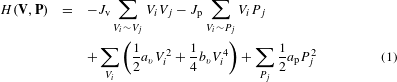The first two sums are vortex–vortex and vortex–pillar interactions with strengths Jv, Jp < 0, where denotes adjacent lattice pairs. The last two sums are individual vortex and pillar circulation potentials. Vortices must be subject to a quartic potential function with bv > 0 to allow for a potentially double-welled potential if av < 0, encoding the observed symmetry breaking into spontaneous circulation in the absence of other interactions15,27. In contrast, our data analysis implies that pillar circulations are sufficiently described by a quadratic potential of strength ap > 0 (Supplementary Fig. 4 and Supplementary Section 4). To account for the experimentally observed spin fluctuations (Fig. 1i and Supplementary Fig. 1), we model the dynamics of the lattice fields V and P through the coupled stochastic differential equations (SDEs)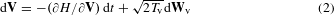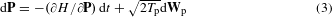where Wv and Wp are vectors of uncorrelated Wiener processes representing intrinsic and thermal fluctuations. The overdamped dynamics in equations (2) and (3) neglects dissipative Onsager-type cross-couplings, as the dominant contribution to friction stems from the nearby no-slip PDMS boundaries (Supplementary Section 7). The parameters Tv and Tp set the strength of random perturbations from energy-minimizing behaviour. In the equilibrium limit when Tv = Tp = T, the stationary statistics of the solutions of equations (2) and (3) obey the Boltzmann distribution eH/T. We inferred all seven parameters of the full SDE model for each experiment by linear regression on a discretization of the SDEs (Supplementary Fig. 2 and Supplementary Section 2). The differing sublattice temperatures TvTp found show that the system is not in thermodynamic equilibrium owing to its active microscopic constituents (Supplementary Fig. 2). Instead, the system is in a pseudo-equilibrium statistical steady state (Supplementary Section 1), which we will soon show can be reduced to an equilibrium-like description. As a cross-validation, we fitted appropriate functions of gap width w to these estimates and simulated the resulting SDE model over a range of w on a 6 × 6 lattice concordant with the observations (Supplementary Section 3). The agreement between experimental data and the numerically obtained vortex–vortex correlation χ(w) supports the validity of the double-lattice model and its underlying approximations (Fig. 1j).

To reconnect with the classical 2D Ising model and understand better the experimentally observed phase transition, we project the Hamiltonian (1) onto an effective square lattice model by making a mean-field assumption for the pillar circulations. In the experiments, Pi is linearly correlated with the average spin of its vortex neighbours [Pi]V = (1/4)${\Sigma }_{j:{V}_{j}\sim {P}_{i}}$Vj, with a constant of proportionality −α < 0 only weakly dependent on gap width (Supplementary Fig. 4 and Supplementary Section 4). Replacing effectively Pi → − α[Pi]V as a mean-field variable in the model eliminates all pillar circulations, yielding a standard quartic LFT for V (see Supplementary Section 4 for a detailed derivation). The mean-field dynamics are then governed by the reduced SDE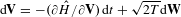with effective temperature TTv + 4TpJp2/ap2 and energy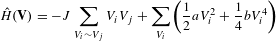which has steady-state probability density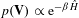with β = 1/T, and where a = av − 4Jp2/ap and b = bv. Note that in the limit a → − and b → + with a/b fixed, the classical two-state Ising model is recovered by identifying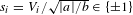. The reduced coupling constant J relates to those of the double-lattice model (Jv, Jp) in the thermodynamic limit as JJv − (1/2)αJp (Supplementary Section 4), making manifest how competition between Jv and Jp can result in both antiferromagnetic (|Jv| > (1/2)α |Jp|) or ferromagnetic (|Jv| < (1/2)α |Jp|) behaviour. We estimated βJ, βa and βb for each experiment by directly fitting the effective one-spin potential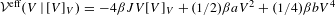via the log-likelihood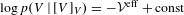(Fig. 2, Supplementary Fig. 5 and Supplementary Section 5). These estimates match those obtained independently using SDE regression methods (Fig. 2a–c and Supplementary Section 5), and show the transition from antiferromagnetic interaction (βJ < 0) to ferromagnetic interaction (βJ > 0) at wcrit (Fig. 2a). As the gap width increases, the energy barrier to spin change falls (Fig. 2b) and the magnitude of the lowest energy spin decreases (Fig. 2c) as a result of weakening confinement within each cavity, visible as a flattening of the one-spin effective potential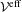(Fig. 2d–f and Supplementary Fig. 5).Figure 2: Best-fit mean-field LFT model captures the phase transition in the square lattice.

Experiments on lattices of different symmetry groups lend further insight into the competition between edge currents and bulk flow. Unlike their square counterparts, triangular lattices cannot support antiferromagnetic states without frustration. Therefore, ferromagnetic order should be enhanced in a triangular bacterial spin lattice. This is indeed observed in our experiments: at moderate gap size w 18 μm, we found exclusively a highly robust ferromagnetic phase of either handedness (Fig. 3a, b, d and Supplementary Movie 4), reminiscent of quantum vortex lattices in Bose–Einstein condensates30. At comparable gap size, the spin correlation is approximately four to eight times larger than in the square lattice. Increasing the gap size beyond 20 μm eventually destroys the spontaneous circulation within the cavities and a disordered state prevails (Fig. 3c, d), with a sharper transition than for the square lattices (Fig. 1j). Conversely, a 1D line lattice exclusively exhibits antiferromagnetic order as the suspension is unable to maintain the very long uniform edge currents that would be necessary to sustain a ferromagnetic state (Supplementary Fig. 6 and Supplementary Section 6). These results manifest the importance of lattice geometry and dimensionality for vortex ordering in bacterial spin lattices, in close analogy with their electromagnetic counterparts.

Understanding the ordering principles of microbial matter is a key challenge in active materials design13, quantitative biology and biomedical research. Improved prevention strategies for pathogenic biofilm formation, for example, will require detailed knowledge of how bacterial flows interact with complex porous surface structures to create the stagnation points at which biofilms can nucleate. Our study shows that collective excitations in geometrically confined bacterial suspensions can spontaneously organize in phases of magnetic order that can be robustly controlled by edge currents. These results demonstrate fundamental similarities with a broad class of widely studied quantum systems17,19,30, suggesting that theoretical concepts originally developed to describe magnetism in disordered media could potentially capture microbial behaviours in complex environments. Future studies may try to explore further the range and limits of this promising analogy.

## Methods

### Experiments.

Wild-type Bacillus subtilis cells (strain 168) were grown in Terrific Broth (Sigma). A monoclonal colony was transferred from an agar plate to 25 ml of medium and left to grow overnight at 35 °C on a shaker. The culture was diluted 200-fold into fresh medium and harvested after approximately 5 h, when more than 90% of the bacteria were swimming, as visually verified on a microscope. 10 ml of the suspension was then concentrated by centrifugation at 1,500g for 10 min, resulting in a pellet with volume fraction approximately 20% which was used without further dilution.

The microchambers were made of polydimethyl siloxane (PDMS) bound to a glass coverslip by oxygen plasma etching. These comprised a square, triangular or linear lattice of 18-μm-deep circular cavities with 60 μm between centres, each of diameter 50 μm, connected by 4–25-μm-wide gaps for linear and square lattices (Fig. 1a, e and Supplementary Fig. 6) and 10–25-μm-wide gaps for triangular lattices (Fig. 3a–c). The smallest possible gap size was limited by the fidelity of the etching.

Approximately 5 μl of the concentrated suspension was manually injected into the chamber using a syringe. Both inlets were then sealed to prevent external flow. We imaged the suspension on an inverted microscope (Zeiss, Axio Observer Z1) under bright-field illumination, through a 40× oil-immersion objective. Movies 10 s in length were recorded at 60 f.p.s. on a high-speed camera (Photron Fastcam SA3) at 4 and 8 min after injection. Although the PDMS lattices were typically 15 cavities across, to avoid boundary effects and to attain the pixel density necessary for PIV we imaged a central subregion spanning 6 × 6 cavities for square lattices, 7 × 6 cavities for triangular lattices, and 7 cavities for linear lattices (multiple of which were captured on a single slide).

Fluorescence in Supplementary Movie 3 was achieved by labelling the membranes of a cell subpopulation with fluorophore FM4-64 following the protocol of Lushi et al. 16 The suspension was injected into an identical triangular lattice as in the primary experiments and imaged at 5.6 f.p.s. on a spinning-disc confocal microscope through a 63× oil-immersion objective.

### Analysis.

For each frame of each movie, the bacterial suspension flow field u(x, y, t) was measured by standard particle image velocimetry (PIV) without time averaging, using a customized version of mPIV (http://www.oceanwave.jp/softwares/mpiv). PIV subwindows were 16 × 16 pixels with 50% overlap, yielding 150 vectors per cavity per frame. Cavity regions were identified in each movie by manually placing the centre and radius of the bottom left cavity, measuring vectors to its immediate neighbours, and repeatedly translating to generate the full grid. Pillar edges were then calculated from the cavity grid and the gap width (measured as the minimum distance between adjacent pillars).

The spin Vi(t) of each cavity i at time t is defined as the normalized planar angular momentum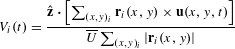where ri(x, y) is the vector from the cavity centre to (x, y), and sums run over all PIV grid points (x, y)i inside cavity i. For each movie, we normalize velocities by the root-mean-square (r.m.s.) suspension velocity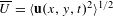, where the average is over all grid points (x, y) and all times t, to account for the effects of variable oxygenation on motility9; we found an ensemble average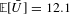μm s−1 with s.d. 3.6 μm s−1 over all experiments. This definition has Vi(t) > 0 for anticlockwise spin and Vi(t) < 0 for clockwise spin. A vortex of radially independent speed—that is,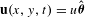, whereis the azimuthal unit vector—has Vi(t) = ±1; conversely, randomly oriented flow has Vi(t) = 0. The average spin–spin correlation χ of a movie is then defined as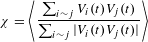where Σij denotes a sum over pairs {i, j} of adjacent cavities and 〈〉 denotes an average over all frames. If all vortices share the same sign, then χ = 1 (ferromagnetism); if each vortex is of opposite sign to its neighbours, then χ = −1 (antiferromagnetism); if the vortices are uniformly random, then χ = 0. Similarly, the circulation Pj(t) about pillar j at time t is defined as the normalized average tangential velocity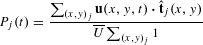where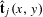is the unit vector tangential to the pillar, and sums run over PIV grid points (x, y)j closer than 5 μm to the pillar j.

Results presented are typically averaged in bins of fixed gap width. All plots with error bars use 3 μm bins, calculated every 1.5 μm (50% overlap), and bins with fewer than five movies were excluded. Error bars denote standard error. Bin counts for square lattices (Figs 1j, k and 2a–c and Supplementary Figs 4 and 7) are 8, 8, 13, 14, 21, 27, 27, 22, 18, 22, 20, 11, 7, 13, 7; bin counts for triangular lattices (Fig. 3d) are 5, 14, 16, 13, 16, 15, 5, 5, 10, 7; and bin counts for linear lattices (Supplementary Fig. 6) are 5, 7, 8, 8, 9, 9, 6, 5, 6, 7, 6, 8, 9, 5, 6, 5, 6.